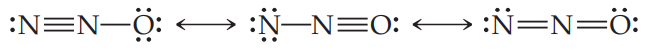×
Get Full Access to Chemistry: The Central Science - 12 Edition - Chapter 8 - Problem 92ae
Get Full Access to Chemistry: The Central Science - 12 Edition - Chapter 8 - Problem 92ae

×

# The following three Lewis structures can be drawn for N2O:ISBN: 9780321696724 27

## Solution for problem 92AE Chapter 8

Chemistry: The Central Science | 12th Edition

• Textbook Solutions
• 2901 Step-by-step solutions solved by professors and subject experts
• Get 24/7 help from StudySoup virtual teaching assistantsChemistry: The Central Science | 12th Edition

4 5 1 295 Reviews
15
5
Problem 92AE

The following three Lewis structures can be drawn for N2O:(a) Using formal charges, which of these three resonance forms is likely to be the most important? (b) The N一N bond length in N2O is 1.12 Å, slightly longer than a typical N☰N bond; and the N一O bond length is 1.19 Å, slightly shorter than a typical N=O bond. (See Table 8.5.) Rationalize these observations in terms of the resonance structures shown previously and your conclusion for part (a).

Step-by-Step Solution:
Step 1 of 3

Chapter 6 : Bond Concepts ( Part 2 ) Bond- Pricing Equation With the Texas Instruments BA-II plus , we have : n = number of periods to maturity I/Y = period interest rate = y P = PV = present value = bond value C = PMT = coupon payment FV = M = face value = par value = future value With the excel , we have : = FV ( Rate, Nper , Pmt , PV , 0/1 ) => calculate the face value = PV (Rate, Nper , Pmt, FV , 0/1 ) => calculate the bond value = RATE ( Nper , Pmt , PV, FV , 0/1) => calculate the period interest rate ( I/Y) = NPER ( Rate, Pmt, PV , FV , 0/1) => calculate the number of periods to maturity = PMT ( rate, nper, pv ,fv , 0/1 ) => calculate coupon payment With 0/1 : Ordinary annuity = 0 ( default ) Annuity Due = 1 Graphical Relationship bet

Step 2 of 3

Step 3 of 3

##### ISBN: 9780321696724

Unlock Textbook Solution